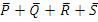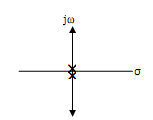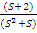# For the circuit shown in the figure the Boolean expression for the output Y in the terms of inputs  P,Q,R and S

1.

2.  P+Q+R+S

3.

4.  (P+Q)(R+S)

4Explanation :
No Explanation available for this question

# In the circuit shown in the figure it is found that the input ac voltage (v) and current i are in phase.the coupling co-efficient is K= where, M is the mutual inductance between the two coils.the value of K and the dot polarity of the coil P-Q are

1.  K=0.25 and dot at P

2.  K=0.5 and dot at P

3.  K=0.25 and dot at Q

4.  K=0.5 and dot at Q

4

K=0.25 and dot at Q

Explanation :
No Explanation available for this question

# consider the circuit shown in the figure if the frequency of the source is 50 Hz,then the value of to which results in a transient free response is

1.  0 ms

2.  1.78 ms

3.  2.71 ms

4.  2.91 ms

4

1.78 ms

Explanation :
No Explanation available for this question

# A three phase thyristor bridge rectifier is used in a HVDC link.The firing angle α as measured from the point of natural commutation is constrained to lie between 5o and 30o.if the dc side current and ac side voltage magnitudes are constant,which of the following statements is true(neglect harmonics in the ac side currents and commutation overlap in your analysis)

1.  reactive power absorbed by the rectifier is maximum when α=5o

2.  reactive power absorbed by the rectifier is maximum when α=30o

3.  reactive power absorbed by the rectifier is maximum when α=15o

4.   reactive power absorbed by the rectifier is maximum when α=10o

4

reactive power absorbed by the rectifier is maximum when α=30o

Explanation :
No Explanation available for this question

# A power system consists of 2 areas (area 1 and area 2)connected by a single tie line.it is required to carry out a load flow study on this system.while entering the network data(connectivity and parameters)is inadvertently left out.if the load flow program is run with this incomplete data.

1.  the load-flow will converge only if the slack bus is specified in area 1

2.  the load-flow will converge only if the slack bus is specified in area 2

3.  the load-flow will converge only if the slack bus is specified in either area 1 or area 2

4.  the load-flow will not converge if only one slack bus is specified.

4

the load-flow will not converge if only one slack bus is specified.

Explanation :
No Explanation available for this question

# A transmission line has a total series reactance of 0.2 pu.Reactive power compensation is applied at the midpoint of the line and it is controlled such that the midpoint voltage of the transmission line is always maintained at 0.98 pu.if voltage at both ends of the line are maintained at 1.0 pu,then the steady state power transfer limit of the transmission line is

1.  9.8 pu

2.  4.9 pu

3.  19.6 pu

4.  5 pu

4

5 pu

Explanation :
No Explanation available for this question

# A generator is connected to a transformer which feeds another transformer through a short feeder.the zero sequence impedance values are expressed in pu on a common base and are indicated in the beiow figure.the thevenin equivalent zero sequence impedance at point B is

1.  0.8+j0.6

2.  0.75+j0.22

3.  0.75+j0.25

4.  1.5+j0.25

4

0.75+j0.22

Explanation :
No Explanation available for this question

# For the system X=X+] u,which of the following statements is true

1.  the system is controllable but unstable

2.  the system is uncontrollable but unstable

3.  the system is controllable but stable

4.  the system is uncontrollable but stable

4

the system is uncontrollable but unstable

Explanation :
No Explanation available for this question

# A unity feedback system has an open loop function,G(s)=.the root locus plot is

1.

2.

3.

4.

4Explanation :
No Explanation available for this question

# The transfer function of the system described by with u as input and y as output is

1.

2.

3.

4.

4